While performing OCR on a photo, we typically extract each character by detecting its bounding rectangle. However, this technique does not apply to cursive scripts where characters are linked with one another.

Let’s consider two cases: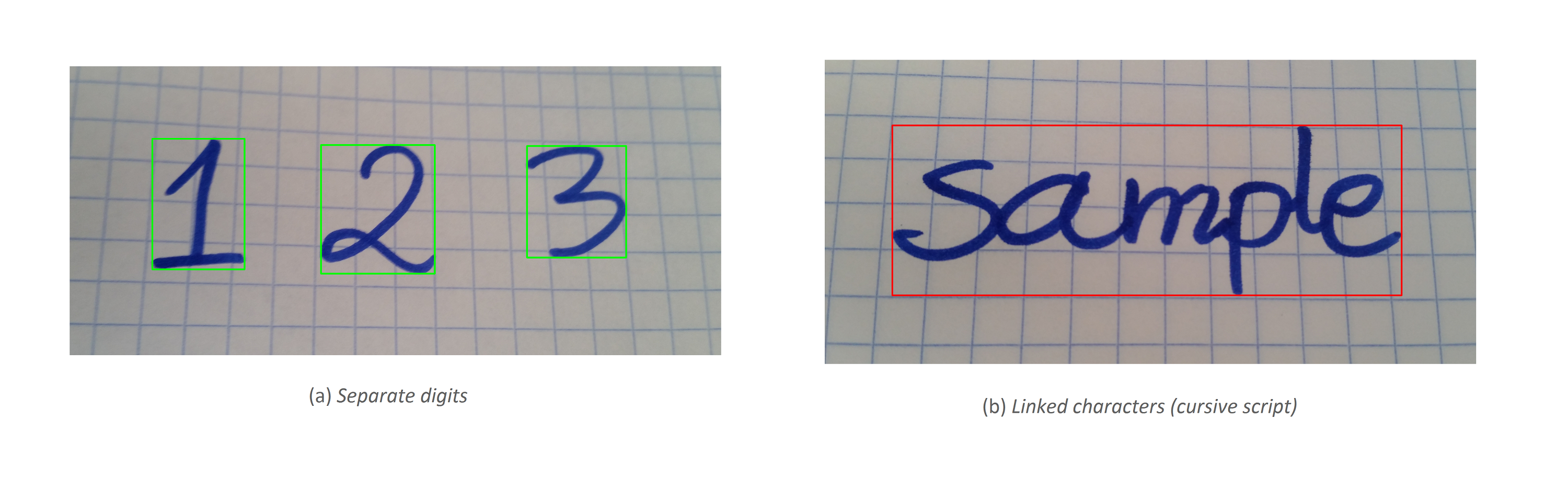In order to separate linked characters shown in illustration (b), our algorithm needs to deal with: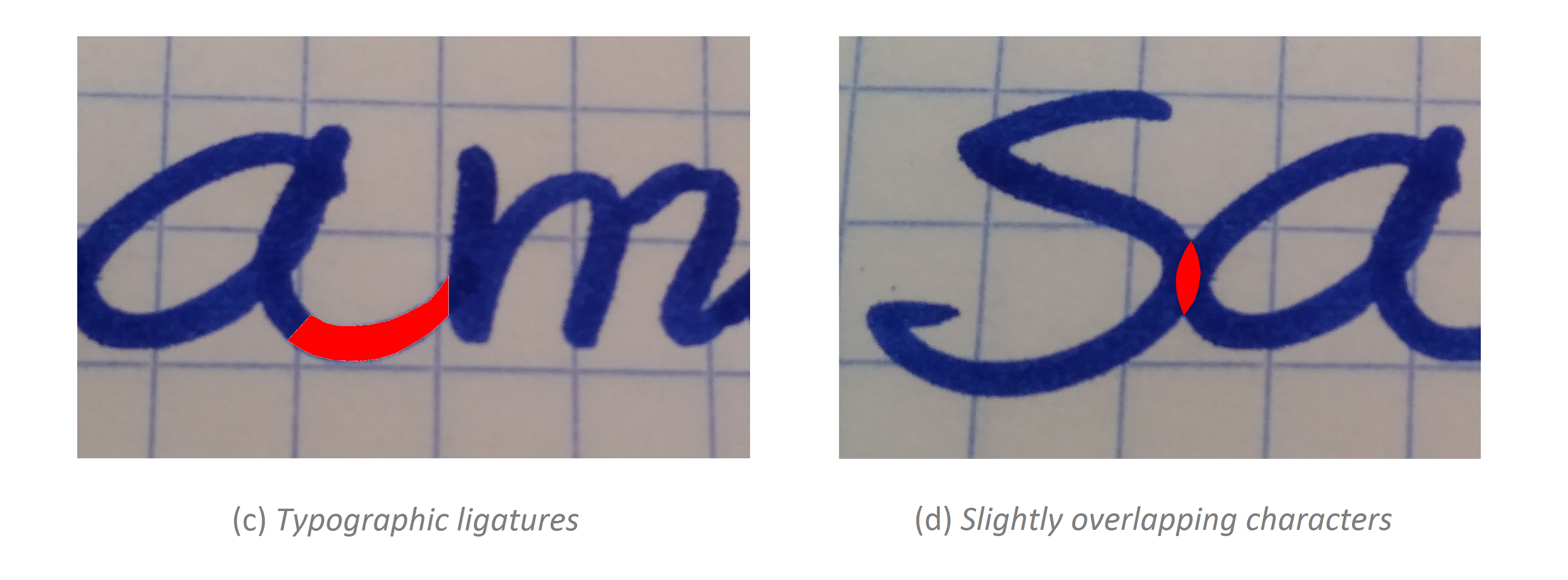### Solution

We propose an algorithm that relies on sliding window and pre-trained classifier.

Pre-trained classifier is capable of classifying all sorts of data (e.g. images) into one (can be multiple) of classes that appeared in training dataset.
Technically, it is an array of numbers that were trained (optimized) in order to minimize so called loss-function. Loss-function tells us how big the total error is after summing up all incorrect guesses made by classifier.

### Training dataset

For our research purposes, we consider dataset containing 30211 samples representing handwritten lowercase letters.

Data description:

• Characters are indicated by black color, whereas background is white.
• Characters are thinned (they are 1 pixel thick).
• Characters are centered within 32x32 square box.

Visualization:### Algorithm

1. Find Outer ROI

We get Outer Region Of Interest by finding the bounding rectangle of linked characters. Such bounding rectangle appears in illustration (b) as marked red.

2. Process Outer ROI

Apply same processing techniques that were applied to training set samples.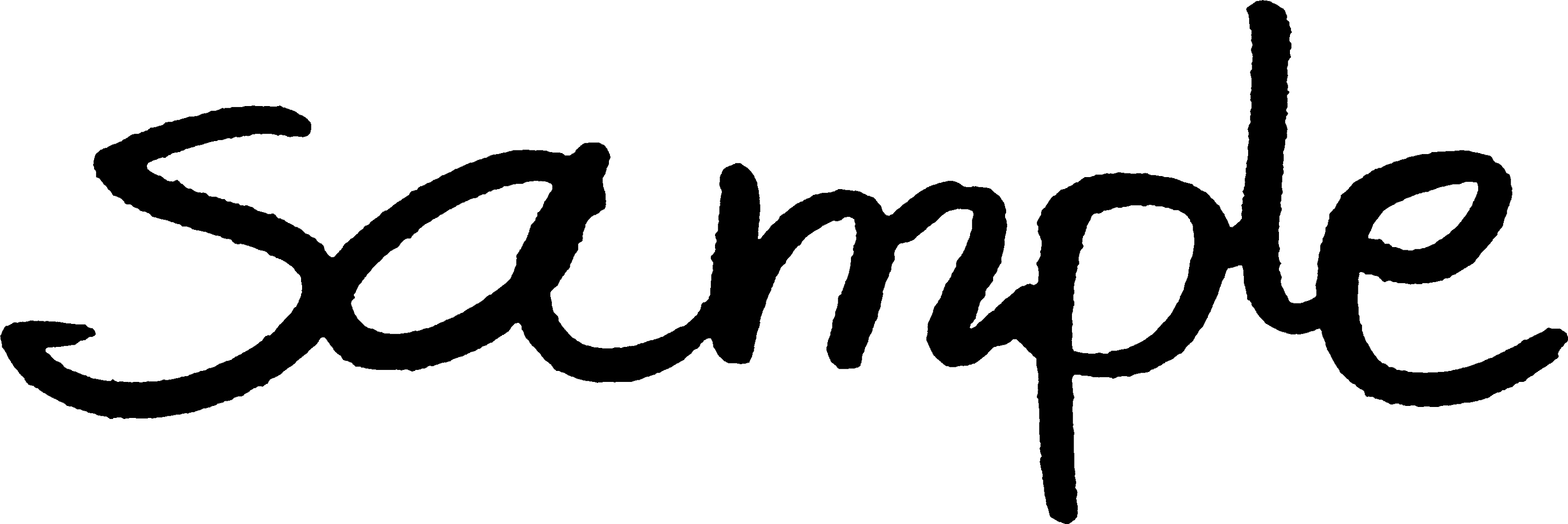3. Initialize sliding window

The initial height of the window is equal to Outer ROI’s height.
The initial width is given by the following condition:
Let $W$ be the width of Outer ROI. Let $w$ be the initial width of sliding window.
Then $w < W$.

Specify stride parameter, which tells how far to the right, we move the sliding window.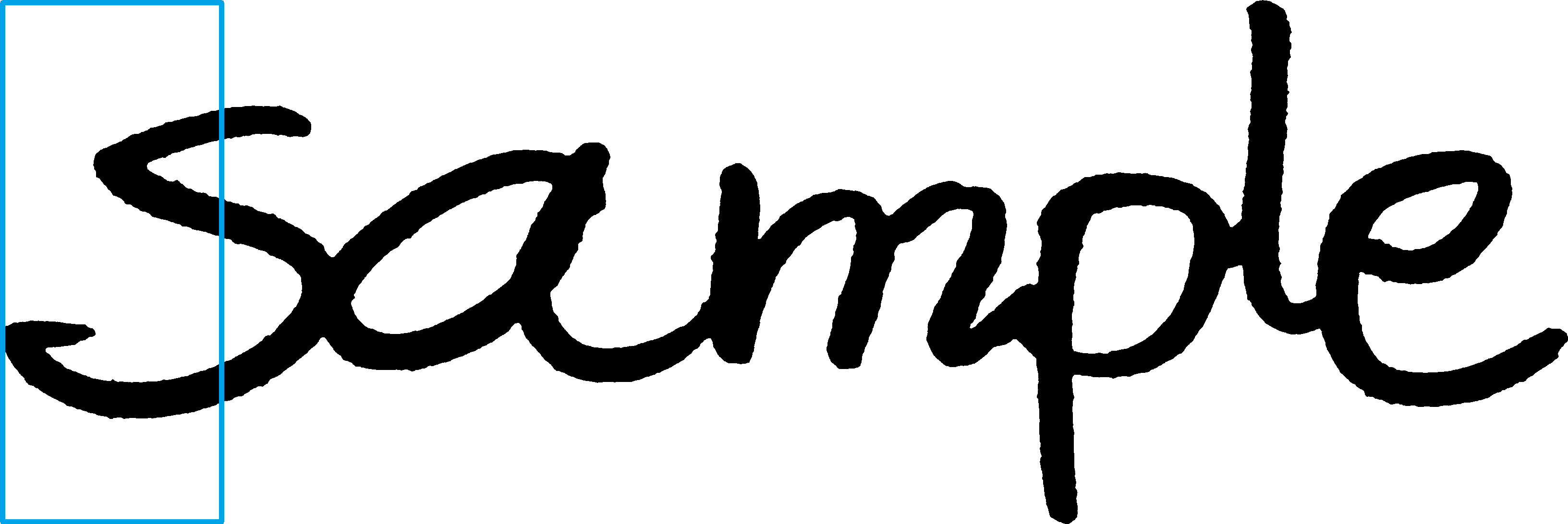4. Find Inner ROI

We get Inner ROI by finding the bounding rectangle of the blob found within the sliding window.

A Blob is a group of connected pixels in an image that share some common property (e.g. grayscale intensity value). In our case, all connected black pixels found within the sliding window are considered as a blob.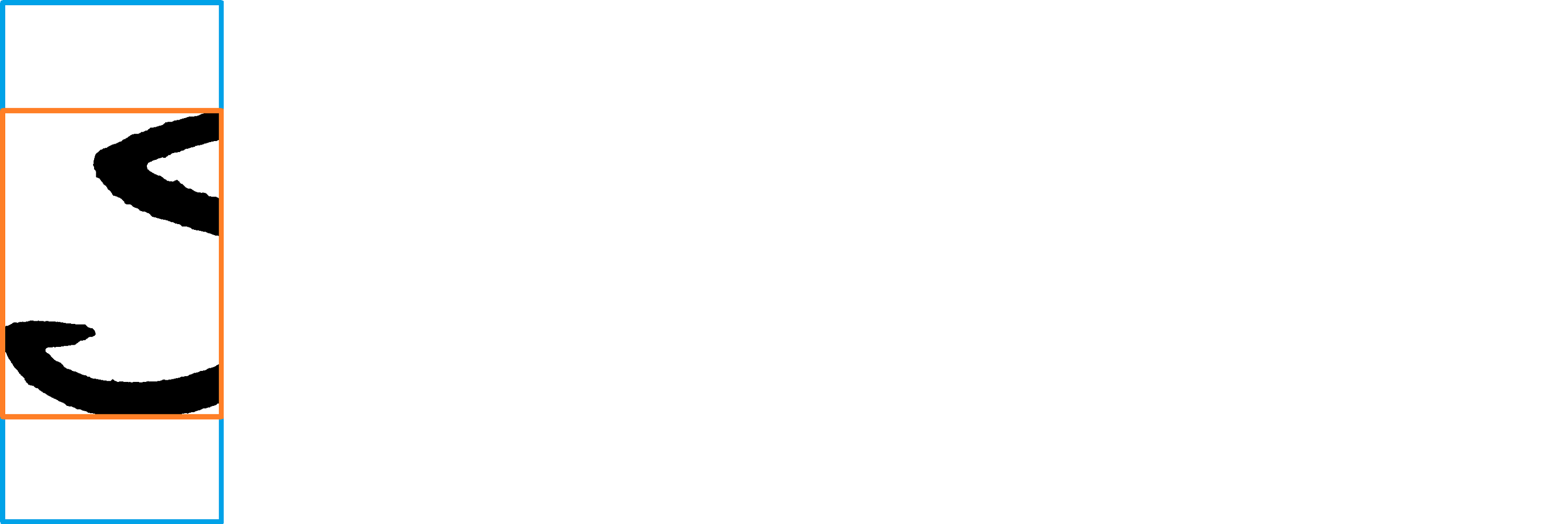5. Process Inner ROI

Fit Inner ROI into a square box, whose sides are equally long to the longer side of the Inner ROI. Center it inside, leaving white space on both sides. Last but not least, downsize the square box to training sample size.

Resulting image is considered as “Standardized Inner ROI”.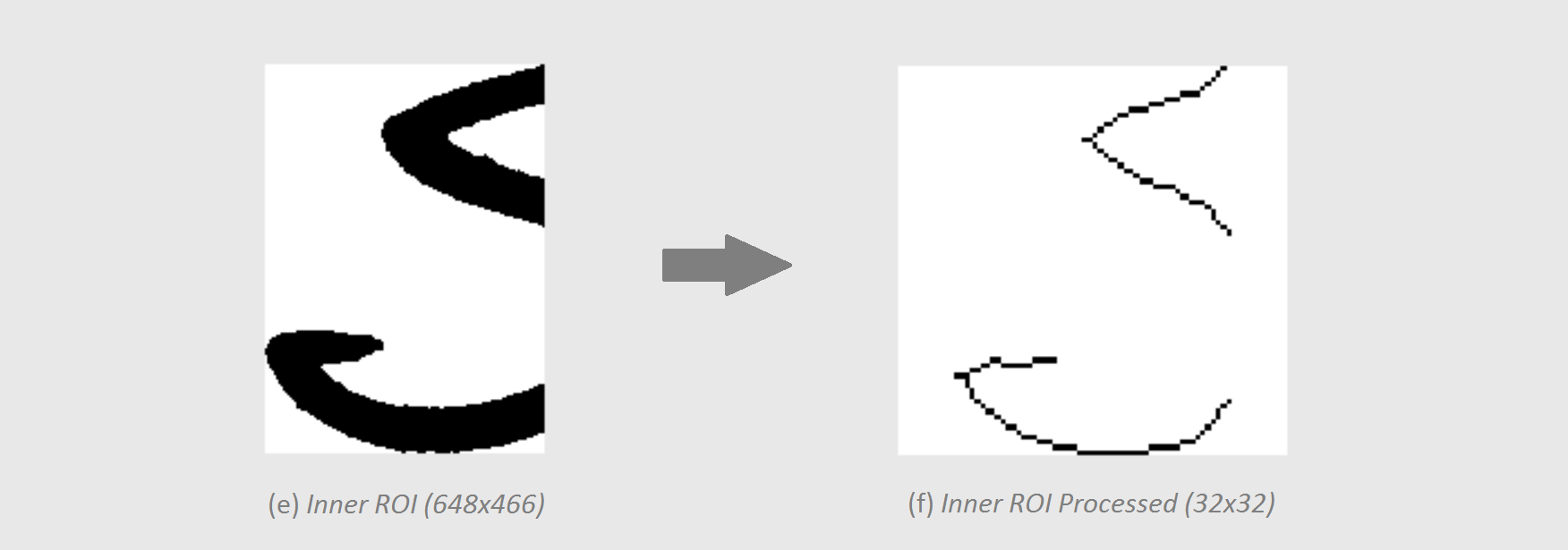6. Run classifier

We employ pre-trained classifier to detect characters within an image, no matter they are linked with one another or slightly overlapping.

Measuring the confidence, the classifier outputs, we might reject sliding window contents as representing a character.

If the highest output confidence is greater than the confidence threshold parameter, then we keep Inner ROI as a candidate for representing a valid letter. Specifically, we keep Inner ROI’s properties, like its coordinates and dimensions.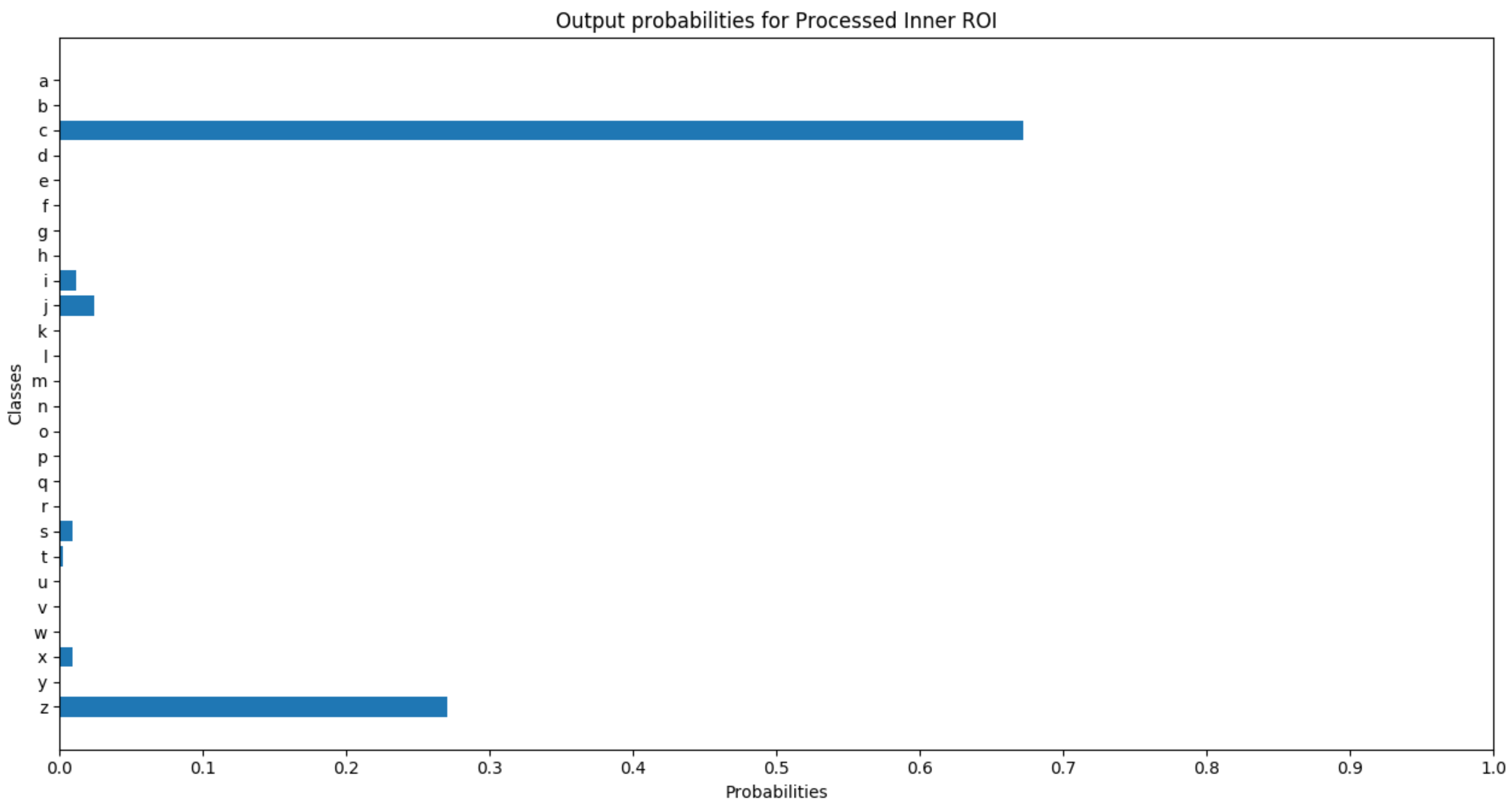7. Move the window

Move sliding window to the right by the number of pixels, specified by the stride parameter.

8. Repeat the process

• If sliding window hasn’t reached the end yet:

Repeat Steps 4-7.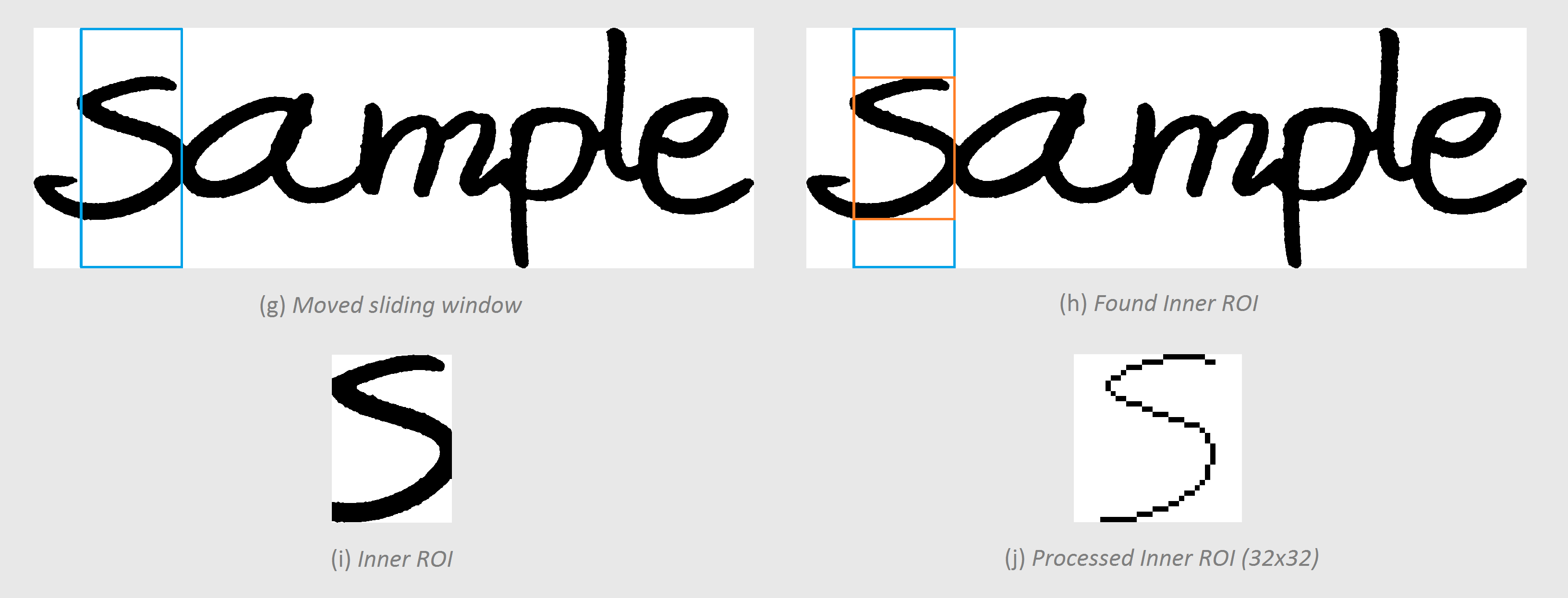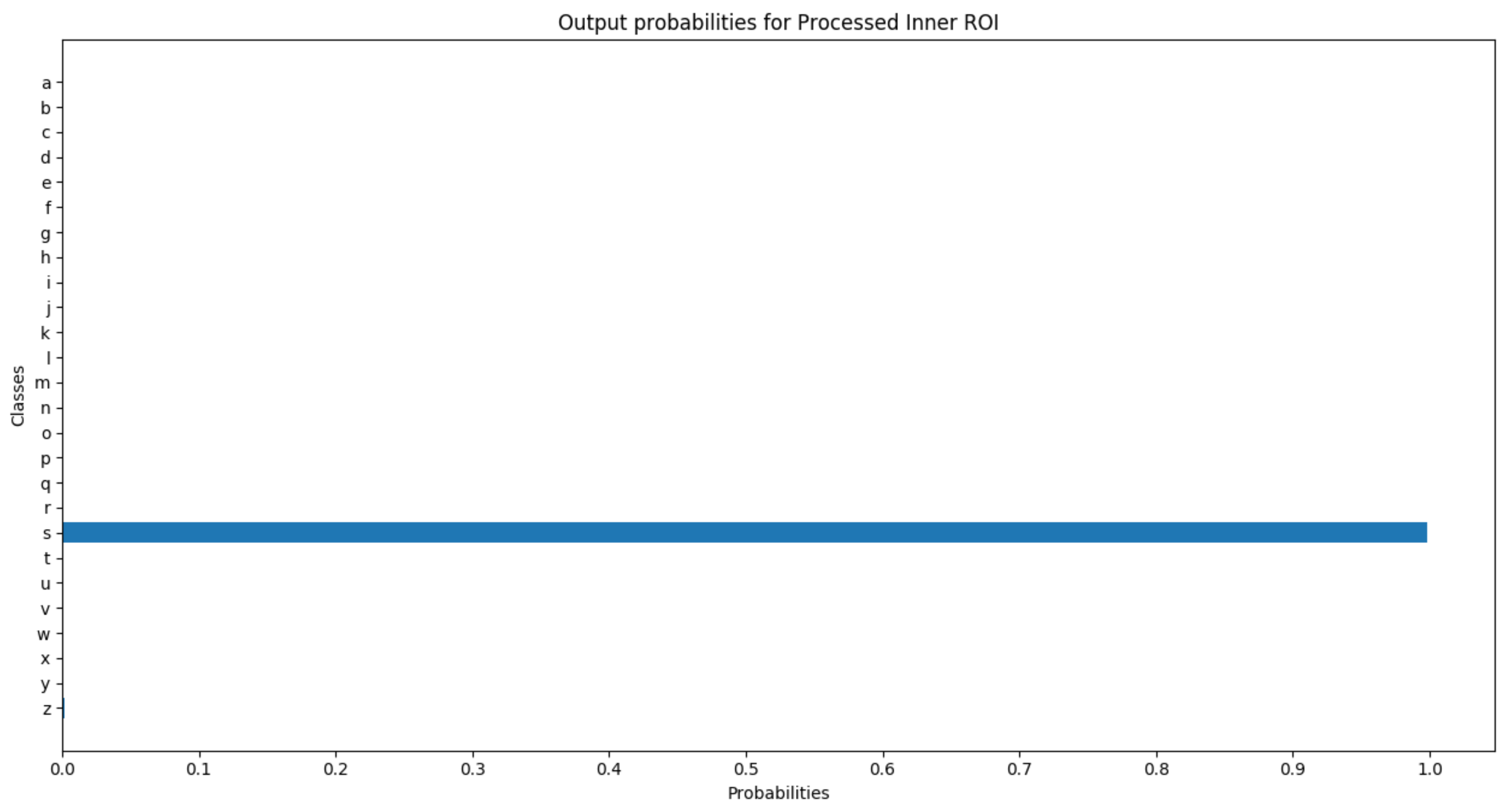• If sliding window has reached the end:

Make sliding window wider, and repeat the process.

• If sliding window’s width is equal to Outer ROI’s width:

Extract characters.

### Extracting characters

Last but not least, we disqualify all candidates that are contained within other candidates.

If overlap of one candidate over the other is smaller than overlap threshold parameter, both candidates are qualified. Where overlap threshold parameter is an arbitrary number greater than 0.